Q-Chem 4.3 User’s Manual

# 11.3 Stand-Alone QM/MM calculations

Q-Chem can perform hybrid quantum mechanics/molecular mechanics (QM/MM) calculations either as a stand-alone program (which is described in this Section) or in conjunction with the Charmm package  (see Section 11.4).

## 11.3.1 Available QM/MM Methods and Features

Three modes of operation are available:

• MM calculations only (no QM)

• QM/MM calculations using a two-layer ONIOM model with mechanical embedding

• QM/MM calculations using the Janus model for electronic embedding

Q-Chem can carry out purely MM calculations, wherein the entire molecular system is described by a MM force field and no electronic structure calculation is performed. The MM force fields available are present are AMBER , CHARMM , and OPLSAA .

As implemented in Q-Chem, the ONIOM model  is a mechanical embedding scheme that partitions a molecular system into two subsystems (layers): an MM subsystem and a QM subsystem. The total energy of an ONIOM system is given by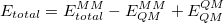(11.14)

where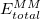is the MM energy of the total system (i.e., QM + MM subsystems),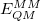is the MM energy of the QM subsystem, and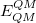is the QM energy of the QM subsystem. MM energies are computed via a specified MM force field, and QM energies are computed via a specified electronic structure calculation.

The advantage of the ONIOM model is its simplicity, which allows for straightforward application to a wide variety of systems. A disadvantage of this approach, however, is that QM subsystem does not interact directly with the MM subsystem. Instead, such interactions are incorporated indirectly, in thecontribution to the total energy. As a result, the QM electron density is not polarized by the electrostatic charges of the MM subsystem.

If the QM/MM interface partitions the two subsystems across a chemical bond, a link atom (hydrogen) must be introduced to act as a cap for the QM subsystem. Currently, Q-Chem supports only carbon link atoms, of atom type 26, 35, and 47 in the CHARMM27 force field.

The Janus model  is an electronic embedding scheme that also partitions the system into MM and QM subsystems, but is more versatile than the ONIOM model. The Janus model in Q-Chem is based upon the “YinYang atom” model of Shao and Kong  . In this approach, the total energy of the system is simply the sum of the subsystem energies,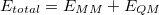(11.15)

The MM subsystem energy,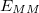, includes van der Waals interactions between QM and MM atoms but not QM/MM Coulomb interactions. Rather,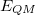includes the direct Coulomb potential between QM atoms and MM atoms as external charges during the QM calculation, thus allowing the QM electron density to be polarized by the MM atoms. Because of this, Janus is particularly well suited (as compared to ONIOM) for carrying out excited-state QM/MM calculations, for excited states of a QM model system embedded within the electrostatic environment of the MM system. Within a Janus calculation, Q-Chem first computeswith the specified force field and then computeswith the specified electronic structure theory.

When the Janus QM/MM partition cuts across a chemical bond, a YinYang atom  is automatically introduced by Q-Chem. This atom acts as a hydrogen cap in the QM calculation, yet also participates in MM interactions. To retain charge neutrality of the total system, the YinYang atom has a single electron and a modified nuclear charge in the QM calculation, equal to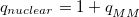(i.e., the charge of a proton plus the charge on the YinYang atom in the MM subsystem).

Because this modified charge will affect the bond containing the YinYang atom, an additional repulsive Coulomb potential is applied between the YinYang atom and its connecting QM atom to maintain a desirable bond length. The additional repulsive Coulomb energy is added to. The YinYang atom can be an atom of any kind, but it is highly recommended to use carbon atoms as YinYang atoms.

Q-Chem’s stand-alone QM/MM capabilities also include the following features:

• Analytic QM/MM gradients are available for QM subsystems described with density functional theory (DFT) or Hartree-Fock (HF) electronic structure theory, allowing for geometry optimizations and QM/MM molecular dynamics.

• Single-point QM/MM energy evaluations are available for QM subsystems described with most post-HF correlated wavefunctions.

• Single-point QM/MM calculations are available for excited states of the QM subsystem, where the latter may be described using CIS, TDDFT, or correlated wavefunction models. Analytic gradients for excited states are available for QM/MM calculations if the QM subsystem is described using CIS.

• Single-point MM or QM/MM energy evaluations are available using periodic boundary conditions with Ewald summation.

• Implicit solvation for both Janus QM/MM calculations as well as MM-only calculations is available using the Polarizable Continuum Models (PCMs) discussed in Section 11.2.2.

• Gaussian blurring of MM external charges is available for Janus QM/MM calculations.

• The user may add new MM atoms types and MM parameters.

• The user may define his/her own force field.

## 11.3.2 Using the Stand-Alone QM/MM Features

### 11.3.2.1 $molecule section To perform QM/MM calculations, the user must assign MM atom types for each atom in the$molecule section. The format for this specification is modeled upon that used by the Tinker molecular modeling package , although the Tinker program is not required to perform QM/MM calculations using Q-Chem. Force field parameters and MM atom type numbers used within Q-Chem are identical to those used Tinker for the AMBER99, CHARMM27, and OPLSAA force fields, and the format of the force field parameters files is also the same.

The $molecule section must use Cartesian coordinates to define the molecular geometry for internal QM/MM calculations; the Z-matrix format is not valid. MM atom types are specified in the$molecule section immediately after the Cartesian coordinates on a line so that the general format for the $molecule section is $molecule
<Charge> <Multiplicity>
<Atom> <X> <Y> <Z> <MM atom type>
. . .
$end  For example, one can define a TIP3P water molecule using AMBER99 atom types, as follows: $molecule
0 1
O        -0.790909    1.149780    0.907453  2001
H        -1.628044    1.245320    1.376372  2002
H        -0.669346    1.913705    0.331002  2002
$end  When the input is specified as above, Q-Chem will determine the MM bond connectivity based on the distances between atoms; if two atoms are sufficiently close, they are considered to be bonded. Occasionally this approach can lead to problems when non-bonded atoms are in close proximity of one another, in which case Q-Chem might classify them as bonded regardless of whether the appropriate MM bond parameters are available. To avoid such a scenario, the user can specify the bonds explicitly by setting the$rem variable USER_CONNECT = TRUE, in which case the $molecule section must have the following format $molecule
<Charge> <Multiplicity>
<Atom> <X> <Y> <Z> <MM atom type> <Bond 1> <Bond 2> <Bond 3> <Bond 4>
. . .
$end  Each <Bond #> is the index of an atom to which <Atom> is bonded. Four bonds must be specified for each atom, even if that atom is connected to fewer than four other atoms. (For non-existent bonds, use zero as a placeholder.) Currently, Q-Chem supports no more than four MM bonds per atom. After setting USER_CONNECT = TRUE, a TIP3P water molecule in the AMBER99 force field could be specified as follows: $molecule
0 1
O        -0.790909    1.149780    0.907453  2001  2 3 0 0
H        -1.628044    1.245320    1.376372  2002  1 0 0 0
H        -0.669346    1.913705    0.331002  2002  1 0 0 0
$end  Explicitly defining the bonds in this way is highly recommended. ### 11.3.2.2$force_field_params section

In many cases, all atoms types (within both the QM and MM subsystems) will be defined by a given force field. In certain cases, however, a particular atom type may not be defined in a given force field. For example, a QM/MM calculation on the propoxide anion might consist of a QM subsystem containing an alkoxide functional group, for which MM parameters do not exist. Even though the alkoxide moiety is described using quantum mechanics, van der Waals parameters are nominally required for atoms within the QM subsystem, which interact with the MM atoms via Lennard-Jones-type interactions.

In such cases, there are four possible options, the choice of which is left to the user’s discretion:

1. Use a similar MM atom type as a substitute for the missing atom type.

2. Ignore the interactions associated with the missing atom type.

3. Define a new MM atom type and associated parameters.

4. Define a new force field.

These options should be applied with care. Option 1 involves selecting an atom type that closely resembles the undefined MM atom. For example, the oxygen atom of an alkoxide moiety could perhaps use the MM atom type corresponding to the oxygen atom of a neutral hydroxyl group. Alternatively, the atom type could be ignored altogether (option 2) by specifying MM atom type 0 (zero). Setting the atom type to zero should be accompanied with setting all four explicit bond connections to placeholders if USER_CONNECT = TRUE. An atom type of zero will cause all MM energies involving that atom to be zero.

The third option in the list above requires the user to specify a $force_field_params section in the Q-Chem input file. This input section can be used to add new MM atom type definitions to one of Q-Chem’s built-in force fields. At a minimum, the user must specify the atomic charge and two Lennard-Jones parameters (radius and well depth,), for each new MM atom type. Bond, angle, and torsion parameters for stretches, bends, and torsions involving the new atom type may also be specified, if desired. The format for the$force_field_params input section is

$force_field_params NumAtomTypes <n> AtomType -1 <Charge> <LJ Radius> <LJ Epsilon> AtomType -2 <Charge> <LJ Radius> <LJ Epsilon> . . . AtomType -n <Charge> <LJ Radius> <LJ Epsilon> Bond <a> <b> <Force constant> <Equilibrium Distance> . . . Angle <a> <b> <c> <Force constant> <Equilibrium Angle> . . . Torsion <a> <b> <c> <d> <Force constant> <Phase Angle> <Multiplicity> . . .$end

The first line in this input section specifies how many new MM atom types appear in this section (<n>). These are specified on the following lines labeled with the AtomType tag. The atom type numbers are required to be negative and to appear in the order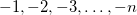. The $molecule section for a water molecule, with user-defined MM parameters for both oxygen and hydrogen, might appear as follows: $molecule
0 1
O        -0.790909    1.149780    0.907453        -1  2 3 0 0
H        -1.628044    1.245320    1.376372        -2  1 0 0 0
H        -0.669346    1.913705    0.331002        -2  1 0 0 0
$end  The remainder of each AtomType line in the$force_field_params section consists of a charge (in elementary charge units), a Lennard-Jones radius (in ), and a Lennard-Jones well depth (, in kcal/mol).

Each (optional) Bond line in the $force_field_params section defines bond-stretching parameters for a bond that contains a new MM atom type. The bond may consist of both atoms <a> and <b> defined an AtomType line, or else <a> may be defined with an AtomType line and <b> defined as a regular atom type for the force field. In the latter case, the label for <b> should be the number of its general van der Waals type. For example, the atom type for a TIP3P oxygen in AMBER99 is 2001, but its van der Waals type is 21, so the latter would be specified in the Bond line. The remaining entries of each Bond line are the harmonic force constant, in kcal/mol/, and the equilibrium distance, in . Similar to the Bond lines, each (optional) Angle line consists of one or more new atom types along with existing van der Waals types. The central atom of the angle is <b>. The harmonic force constant (in units of kcal/mol/degree) and equilibrium bond angle (in degrees) are the final entries in each Bond line. Each (optional) Torsion line consists of one or more new MM atom types along with regular van der Waals types. The connectivity of the four atoms that constitute the dihedral angle is <a><b><c><d>, and the torsional potential energy function is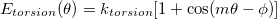(11.16) The force constant (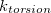) is specified in kcal/mol and the phase angle (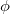) in degrees. The multiplicity () is an integer. ### 11.3.2.3 User-defined force fields Option 4 in the list on page * is the most versatile, and allows the user to define a completely new force field. This option is selected by setting FORCE_FIELD = READ, which tells Q-Chem to read force field parameters from a text file whose name is specified in the$force_field_params section as follows:

$force_field_params Filename <path/filename>$end

Here, <path/filename> is the full (absolute) path and name of the file that Q-Chem will attempt to read for the MM force field. For example, if the user has a file named MyForceField.prm that resides in the path /Users/me/parameters/, then this would be specified as
$force_field_params Filename /Users/me/parameters/MyForceField.prm$end


Within the force field file, the user should first declare various rules that the force field will use, including how van der Waals interactions will be treated, scaling of certain interactions, and the type of improper torsion potential. The rules are declared in the file as follows:

RadiusRule <option>
EpsilonRule <option>
ImptorType <option>
vdw-14-scale <x>
chg-14-scale <x>
torsion-scale <x>


Currently, only a Lennard-Jones potential is available for van der Waals interactions. RadiusRule and EpsilonRule control how to averageand, respectively, between atoms A and B in their Lennard-Jones potential. The options available for both of these rules are Arithmetic [e.g.,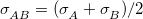] or Geometric [e.g.,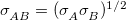]. RadiusSize has options Radius or Diameter, which specify whether the parameteris the van der Waals radius or diameter in the Lennard-Jones potential.

ImptorType controls the type of potential to be used for improper torsion (out-of-plane bending) energies, and has two options: Trigonometric or Harmonic. These options are described in more detail below.

The scaling rules takes a floating point argument <x>. The vdw-14-scale and chg-14-scale rules only affect van der Waals and Coulomb interactions, respectively, between atoms that are separated by three consecutive bonds (atoms 1 and 4 in the chain of bonds). These interaction energies will be scaled by <x>. Similarly, torsion-scale scales dihedral angle torsion energies.

After declaring the force field rules, the number of MM atom types and van der Waals types in the force field must be specified using:

NAtom <n>
Nvdw <n>

where <n> is a positive integer.

Next, the atom types, van der Waals types, bonds, angles, dihedral angle torsion, improper torsions, and Urey-Bradley parameters can be declared in the following format:

Atom 1 <Charge> <vdw Type index> <Optional description>
Atom 2 <Charge> <vdw Type index> <Optional description>
. . .
Atom <NAtom> <Charge> <vdw Type index> <Optional description>
. . .
vdw 1 <Sigma> <Epsilon> <Optional description>
vdw 2 <Sigma> <Epsilon> <Optional description>
. . .
vdw <Nvdw> <Sigma> <Epsilon> <Optional description>
. . .
Bond <a> <b> <Force constant> <Equilibrium Distance>
. . .
Angle <a> <b> <c> <Force constant> <Equilibrium Angle>
. . .
Torsion <a> <b> <c> <d> <Force constant 1> <Phase Angle 1> <Multiplicity 1>
. . .
Improper <a> <b> <c> <d> <Force constant> <Equilibrium Angle> <Multiplicity>
. . .
UreyBrad <a> <b> <c>  <Force constant> <Equilibrium Distance>

The parameters provided in the force field parameter file correspond to a basic MM energy functional of the form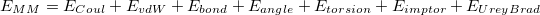(11.17)

Coulomb and van der Waals interactions are computed for all non-bonded pairs of atoms that are at least three consecutive bonds apart (i.e., 1–4 pairs and more distant pairs). The Coulomb energy between atom types 1 and 2 is simply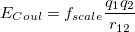(11.18)

where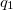and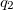are the respective charges on the atoms (specified with <Charge> in elementary charge units) and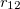is the distance between the two atoms. For 1–4 pairs,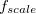is defined with chg-14-scale but is unity for all other valid pairs. The van der Waals energy between two atoms with van der Waals types a and b, and separated by distance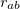, is given by a “6-12” Lennard-Jones potential: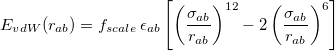(11.19)

Here,is the scaling factor for 1–4 interactions defined with vdw-14-scale and is unity for other valid interactions. The quantities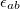and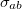are the averages of the parameters of atoms a and b as defined with EpsilonRule and RadiusRule, respectively (see above). The units of <Sigma> are  , and the units of <Epsilon> are kcal/mol. Hereafter, we refer to atoms’ van der Waals types with a, b, c, ... and atoms’ charges with 1,2, 3, ....

The bond energy is a harmonic potential,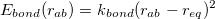(11.20)

where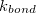is provided by <Force Constant> in kcal/mol/and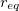by <Equilibrium Distance> in . Note that <a> and <b> in the Bond definition correspond to the van der Waals type indices from the vdw definitions, not the Atom indices.

The bending potential between two adjacent bonds connecting three different atoms (<a>-<b>-<c>) is also taken to be harmonic,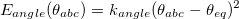(11.21)

Here,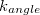is provided by <Force Constant> in kcal/mol/degrees and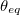by <Equilibrium Angle> in degrees. Again, <a>, <b>, and <c> correspond to van der Waals types defined with vdw.

The energy dependence of the <a>-<b>-<c>-<d> dihedral torsion angle, where <a>, <b>, <c>, and <d> are van der Waals types, is defined by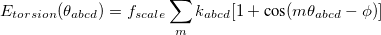(11.22)

Here,is the scaling factor defined by torsion-scale. The force constant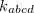is defined with <Force constant> in kcal/mol, and the phase angleis defined with <Phase Angle> in degrees. The summation is over multiplicities,, and Q-Chem supports up to three different values ofper dihedral angle. The force constants and phase angles may depend on, so if more than one multiplicity is used, then <Force constant> <Phase Angle> <Multiplicity> should be specified for each multiplicity. For example, to specify a dihedral torsion between van der Waals types 2–1–1–2, with multiplicities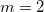and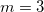, we might have:

Torsion  2  1  1  2  2.500  180.0  2 1.500 60.0 3


Improper torsion angle energies for four atoms <a>-<b>-<c>-<d>, where <c> is the central atom, can be computed in one of two ways, as controlled by the ImptorType rule. If ImptorType is set to Trigonometric, then the improper torsion energy has a functional form similar to that used for dihedral angle torsions: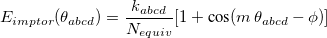(11.23)

Here,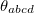is the out-of-plane angle of atom <c>, in degrees, andis the force constant defined with <Force Constant>, in kcal/mol. The phaseand multiplicityneed to be specified in the Improper declaration, although the definition of an improper torsion suggests that these values should be set to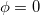and. The quantity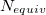accounts for the number of equivalent permutations of atoms <a>, <b>, and <d>, so that the improper torsion angle is only computed once. If ImptorType is set to Harmonic, then in place of Eq. eq:ImpTor_Trig, the following energy function is used: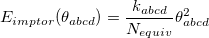(11.24)

The Urey-Bradley energy, which accounts for a non-bonded interaction between atoms <a> and <c> that are separated by two bonds (i.e., a 1-3 interaction through <a>-<b>-<c>), is given by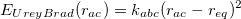(11.25)

The distance in  between atoms <a> and <c> is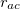, the equilibrium distanceis provided by <Equilibrium Distance> in , and the force constant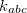is provided by <Force Constant> in kcal/mol/.

A short example of a valid text-only file defining a force field for a flexible TIP3P water could be as follows:

//-- Force Field Example --//

// -- Rules -- //
EpsilonRule Geometric
ImptorType Trigonometric
vdw-14-scale 1.0
chg-14-scale 0.8
torsion-scale 0.5

// -- Number of atoms and vdw to expect -- //
NAtom 2
Nvdw 2

// -- Atoms -- //
Atom 1 -0.8340  2  TIP3P Oxygen
Atom 2  0.4170  1  TIP3P Hydrogen

// -- vdw -- //
vdw 1  0.0000  0.0000 H parameters
vdw 2  1.7682  0.1521 O parameters

// -- Bond -- //
Bond 1 2  553.0  0.9572

// -- Angle -- //
Angle 1 2 1  100.0  104.52

Lines that do not begin with one of the keywords will be ignored, and have been used here as comments.

### 11.3.2.4 $qm_atoms and$forceman sections

For QM/MM calculations (but not for purely MM calculations) the user must specify the QM subsystem using a $qm_atoms input section, which assumes the following format: $qm_atoms
<QM atom 1 index> <QM atom 2 index> . . .
. . .
<QM atom n index>
$end  Multiple indices can appear on a single line and the input can be split across multiple lines. Each index is an integer corresponding to one of the atoms in the$molecule section, beginning at 1 for the first atom in the $molecule section. Link atoms for the ONIOM model and YinYang atoms for the Janus model are not specified in the$qm_atoms section, as these are inserted automatically whenever a bond connects a QM atom and an MM atom.

Q-Chem 4.2.2 and later versions also support, for example

$qm_atoms 18:31 35$end

which specifies 15 QM atoms (atoms 18 through 31; atom 35).

For Janus QM/MM calculations, there are several ways of dealing with van der Waals interactions between the QM and MM atoms. By default, van der Waals interactions are computed for all QM–MM and MM–MM atom pairs but not for QM–QM atom pairs. In some cases, the user may prefer not to neglect the van der Waals interactions between QM–QM atoms, or the user may prefer to neglect any van der Waals interaction that involves a QM atom. Q-Chem allows the user this control via two options in the $forceman section. To turn on QM–QM atom van der Waals interactions, the user should include the following in their input: $forceman
QM-QMvdw
$end  Similarly, to turn off all van der Waals interactions with QM atoms, the following should be included: $forceman
NoQM-QMorQM-MMvdw
$end  ### 11.3.2.5 Periodic Boundary Conditions Periodic boundary conditions (using Ewald summation for the long-range Coulomb interactions) can be used in conjunction with both MM-only calculations and QM/MM calculations. The approach is based off of the work of Nam et al.  and (independently) Riccardi et al. , as implemented in both the AMBER  and CHARMM [658, 660] programs. These approaches use Mulliken charges to represent the periodic images of the wavefunction, and while suitable for semi-empirical calculations with minimal basis sets, instabilities in the Mulliken charges for extended basis sets lead to SCF convergence failure in the QM/MM-Ewald calculations . The implementation in Q-Chem thus allows for the use of CHELPG charges to represent the image wavefunctions, affording an algorithm that is stable in extended basis sets . The efficiency of the Ewald summation is governed by the parameter,, that controls the partition of the Coulomb potential into short- and long-range components, and in the QM/MM-Ewald method there are separate values offor the QM and MM portions of the calculations. Improper selection of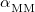and/or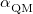can greatly increase the computational time, and the choices that are optimal for MM calculations need not be optimal for QM/MM calculations . The cost of the MM Ewald summation scales as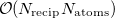, where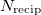is the number of reciprocal-space lattice vectors that is used for the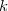-space sum. The QM portion of the calculation scales as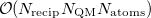, where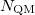is the number of QM atoms (whereas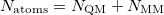is the total number of atoms). The MM Ewald parameter is thus selected to minimize the amount of work that is done in real space. The optimal value, which is typically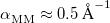, can be found by solving the equation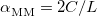(11.26) where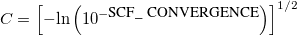(11.27) and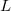is the length of the simulation cell. (Only cubic simulation cells are available at present.) In contrast, the parametershould be selected to minimize the total number of vectors in both real and reciprocal space. The optimal value, which is often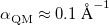, is determined by solving the equation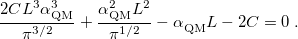(11.28) To perform an MM- or QM/MM-Ewald job, one must set MM_SUBTRACTIVE = TRUE in the$rem section, but otherwise job control is largely done through the $forceman section. The following variables must be set for every type of Ewald calculation. • The keyword Ewald will turn on Ewald summation. • The keyword alpha should be followed by a value for the MM Ewald parameter and then the QM Ewald parameter. (The latter must be set even for MM-only jobs.) • Box_length specifies the side length of the cubic simulation cell, in . The following parameters are optional for further job control. • Dielectric specifies a dielectric constant for the surrounding medium, which appears in the “dipole term” of Ewald summation (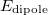in Ref. ). If no value is set, the dielectric constant is set to infinity, corresponding to “tin foil" boundary conditions. • The keyword Ewald_SCF_thresh_on, followed by a real number, causes Q-Chem to wait until the DIIS error falls below the specified value before adding the Ewald correction to the Fock matrix, thus obviating the sometimes-costly Ewald correction in early SCF cycles. (The default value is 1.0, which turns on Ewald summation immediately in most cases) A short example of a$forceman section using Ewald summation could be as follows:

$forceman ewald alpha 0.35 0.1 box_length 15.00 dielectric 88.0 mm_read_scratch ewald_scf_thresh_on 0.0001$end


## 11.3.3 Additional Job Control Variables

A QM/MM job is requested by setting the $rem variables QM_MM_INTERFACE and FORCE_FIELD. Also required are a$qm_atoms input section and appropriate modifications to the $molecule section, as described above. Additional job control variables are detailed here. QM_MM_INTERFACE  Enables internal QM/MM calculations. TYPE:  STRING DEFAULT:  NONE OPTIONS:  MM Molecular mechanics calculation (i.e., no QM region) ONIOM QM/MM calculation using two-layer mechanical embedding JANUS QM/MM calculation using electronic embedding RECOMMENDATION:  The ONIOM model and Janus models are described above. Choosing MM leads to no electronic structure calculation. However, when using MM, one still needs to define the$rem variables BASIS and EXCHANGE in order for Q-Chem to proceed smoothly.

FORCE_FIELD
 Specifies the force field for MM energies in QM/MM calculations.

TYPE:
 STRING

DEFAULT:
 NONE

OPTIONS:
 AMBER99 AMBER99 force field CHARMM27 CHARMM27 force field OPLSAA OPLSAA force field

RECOMMENDATION:
 None.

CHARGE_CHARGE_REPULSION
 The repulsive Coulomb interaction parameter for YinYang atoms.

TYPE:
 INTEGER

DEFAULT:
 550

OPTIONS:Use Q =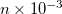RECOMMENDATION:
 The repulsive Coulomb potential maintains bond lengths involving YinYang atoms with the potential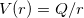. The default is parameterized for carbon atoms.

GAUSSIAN_BLUR
 Enables the use of Gaussian-delocalized external charges in a QM/MM calculation.

TYPE:
 LOGICAL

DEFAULT:
 FALSE

OPTIONS:
 TRUE Delocalizes external charges with Gaussian functions. FALSE Point charges

RECOMMENDATION:
 None

GAUSS_BLUR_WIDTH
 Delocalization width for external MM Gaussian charges in a Janus calculations.

TYPE:
 INTEGER

DEFAULT:
 NONE

OPTIONS:Use a width of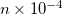.

RECOMMENDATION:
 Blur all MM external charges in a QM/MM calculation with the specified width. Gaussian blurring is currently incompatible with PCM calculations. Values of 1.0–2.0  are recommended in Ref. Das:2002.

MODEL_SYSTEM_CHARGE
 Specifies the QM subsystem charge if different from the $molecule section. TYPE:  INTEGER DEFAULT:  NONE OPTIONS:The charge of the QM subsystem. RECOMMENDATION:  This option only needs to be used if the QM subsystem (model system) has a charge that is different from the total system charge. MODEL_SYSTEM_MULT  Specifies the QM subsystem multiplicity if different from the$molecule section.

TYPE:
 INTEGER

DEFAULT:
 NONE

OPTIONS:The multiplicity of the QM subsystem.

RECOMMENDATION:
 This option only needs to be used if the QM subsystem (model system) has a multiplicity that is different from the total system multiplicity. ONIOM calculations must be closed shell.

USER_CONNECT
 Enables explicitly defined bonds.

TYPE:
 STRING

DEFAULT:
 FALSE

OPTIONS:
 TRUE Bond connectivity is read from the $molecule section FALSE Bond connectivity is determined by atom proximity RECOMMENDATION:  Set to TRUE if bond connectivity is known, in which case this connectivity must be specified in the$molecule section. This greatly accelerates MM calculations.

MM_SUBTRACTIVE
 Specifies whether a subtractive scheme is used in the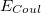, Eq. eq:ECoul, portion of the calculation.

TYPE:
 LOGICAL

DEFAULT:
 FALSE

OPTIONS:
 FALSE Only pairs that are not 1-2, 1-3, or 1-4 pairs are used. TRUE All pairs are calculated, and then the pairs that are double counted (1-2, 1-3, and 1-4) are subtracted out.

RECOMMENDATION:
 When running QM/MM or MM calculations there is not recommendation. When running a QM/MM Ewald calculation the value must be set to TRUE.

## 11.3.4 QM/MM Examples

• QM/MM Example 1

Features of this job:

• Geometry optimization using ONIOM mechanical embedding.

• MM region (water 1) described using OPLSAA.

• QM region (water 2) described using PBE0/6-31G*.

• $molecule input section contains user-defined MM bonds. A zero is used as a placeholder if there are no more connections. Example 11.252 ONIOM optimization of water dimer. $rem
method                  pbe0
basis                   6-31G*
qm_mm_interface         oniom
force_field             oplsaa
user_connect            true
jobtype                 opt
molden_format           true
$end$qm_atoms
4 5 6
$end$molecule
0 1
O        -0.790909    1.149780    0.907453  186  2  3  0  0
H        -1.628044    1.245320    1.376372  187  1  0  0  0
H        -0.669346    1.913705    0.331002  187  1  0  0  0
O         1.178001   -0.686227    0.841306  186  5  6  0  0
H         0.870001   -1.337091    1.468215  187  4  0  0  0
H         0.472696   -0.008397    0.851892  187  4  0  0  0
$end  • QM/MM Example 2 Features of this job: • Janus electronic embedding with a YingYang link atom (the glycosidic carbon at the C1position of the deoxyribose). • MM region (deoxyribose) is described using AMBER99. • QM region (adenine) is described using HF/6-31G*. • The first 5 electronically excited states are computed with CIS. MM energy interactions between a QM atom and an MM atom (e.g., van der Waals interactions, as well as angles involving a single QM atom) are assumed to be the same in the excited states as in the ground state. •$molecule input section contains user-defined MM bonds.

• Gaussian-blurred charges are used on all MM atoms, with a width set to 1.5 .

Example 11.253  Excited-state single-point QM/MM calculation on deoxyadenosine.

$rem method cis basis 6-31G* qm_mm_interface janus user_connect true force_field amber99 gaussian_blur true gauss_blur_width 15000 cis_n_roots 5 cis_triplets false molden_format true print_orbitals true$end

$qm_atoms 18 19 20 21 22 23 24 25 26 27 28 29 30 31$end

$molecule 0 1 O 0.000000 0.000000 0.000000 1244 2 9 0 0 C 0.000000 0.000000 1.440000 1118 1 3 10 11 C 1.427423 0.000000 1.962363 1121 2 4 6 12 O 1.924453 -1.372676 1.980293 1123 3 5 0 0 C 2.866758 -1.556753 0.934073 1124 4 7 13 18 C 2.435730 0.816736 1.151710 1126 3 7 8 14 C 2.832568 -0.159062 0.042099 1128 5 6 15 16 O 3.554295 1.211441 1.932365 1249 6 17 0 0 H -0.918053 0.000000 -0.280677 1245 1 0 0 0 H -0.520597 -0.885828 1.803849 1119 2 0 0 0 H -0.520597 0.885828 1.803849 1120 2 0 0 0 H 1.435560 0.337148 2.998879 1122 3 0 0 0 H 3.838325 -1.808062 1.359516 1125 5 0 0 0 H 1.936098 1.681209 0.714498 1127 6 0 0 0 H 2.031585 -0.217259 -0.694882 1129 7 0 0 0 H 3.838626 0.075227 -0.305832 1130 7 0 0 0 H 4.214443 1.727289 1.463640 1250 8 0 0 0 N 2.474231 -2.760890 0.168322 1132 5 19 27 0 C 1.538394 -2.869204 -0.826353 1136 18 20 28 0 N 1.421481 -4.070993 -1.308051 1135 19 21 0 0 C 2.344666 -4.815233 -0.582836 1134 20 22 27 0 C 2.704630 -6.167666 -0.619591 1140 21 23 24 0 N 2.152150 -7.057611 -1.455273 1142 22 29 30 0 N 3.660941 -6.579606 0.239638 1139 22 25 0 0 C 4.205243 -5.691308 1.066416 1138 24 26 31 0 N 3.949915 -4.402308 1.191662 1137 25 27 0 0 C 2.991769 -4.014545 0.323275 1133 18 21 26 0 H 0.951862 -2.033257 -1.177884 1145 19 0 0 0 H 2.449361 -8.012246 -1.436882 1143 23 0 0 0 H 1.442640 -6.767115 -2.097307 1144 23 0 0 0 H 4.963977 -6.079842 1.729564 1141 25 0 0 0$end


• QM/MM Example 3

Features of this job:

• An MM-only calculation. BASIS and EXCHANGE need to be defined, in order to prevent a crash, but no electronic structure calculation is actually performed.

• All atom types and MM interactions are defined in $force_field_params using the CHARMM27 force field. Atomic charges, equilibrium bond distances, and equilibrium angles have been extracted from a HF/6-31G* calculation, but the force constants and van der Waals parameters are fictitious values invented for this example. • Molecular dynamics is propagated for 10 steps within a microcanonical ensemble (NVE), which is the only ensemble available at present. Initial velocities are sampled from a Boltzmann distribution at 400 K. Example 11.254 MM molecular dynamics with user-defined MM parameters. $rem
basis           sto-3g
method          hf
qm_mm_interface MM
force_field     charmm27
user_connect    true
jobtype         aimd
time_step       42
aimd_steps      10
aimd_init_veloc thermal
aimd_temp       400
$end$molecule
-2 1
C    0.803090    0.000000    0.000000  -1  2  3  6  0
C   -0.803090    0.000000    0.000000  -1  1  4  5  0
H    1.386121    0.930755    0.000000  -2  1  0  0  0
H   -1.386121   -0.930755    0.000000  -2  2  0  0  0
H   -1.386121    0.930755    0.000000  -2  2  0  0  0
H    1.386121   -0.930755    0.000000  -2  1  0  0  0
$end$force_field_params
NumAtomTypes 2
AtomType -1  -0.687157  2.0000  0.1100
AtomType -2  -0.156422  1.3200  0.0220
Bond     -1  -1  250.00  1.606180
Bond     -1  -2  300.00  1.098286
Angle    -2  -1  -2  50.00  115.870
Angle    -2  -1  -1  80.00  122.065
Torsion  -2  -1  -1  -2  2.500  180.0  2
$end  Further examples of QM/MM calculations can be found in the$QC/samples directory, including a QM/MM/PCM example, QMMMPCM_crambin.in. This calculation consists of a protein molecule (crambin) described using a force field, but with one tyrosine side chain described using electronic structure theory. The entire QM/MM system is placed within an implicit solvent model, of the sort described in Section 11.2.2.